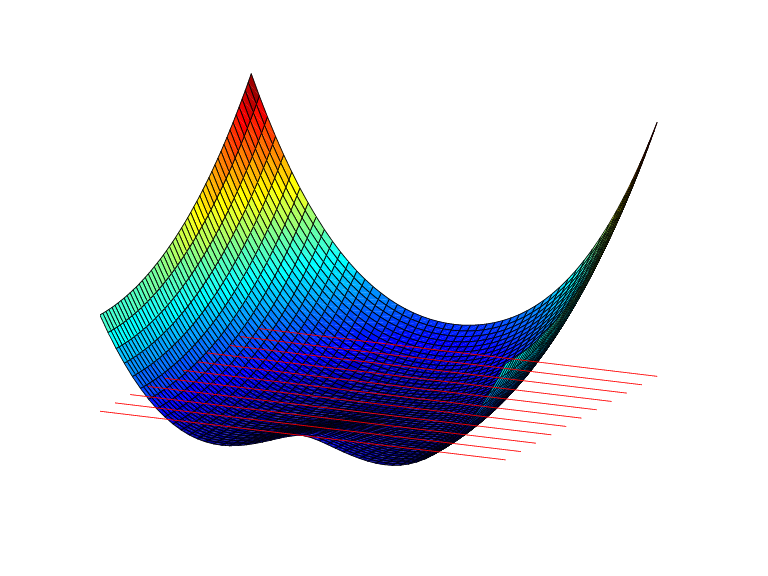# stmak

Put together function in stform

## Syntax

```stmak(centers,coefs) st = stmak(centers,x,type) st = stmak(centers,coefs,type,interv) ```

## Description

`stmak(centers,coefs) ` returns the stform of the function f given by

`$f\left(x\right)=\sum _{j=1}^{n}\text{coefs}\left(:,j\right)\cdot \psi \left(x-\text{centers}\left(:,j\right)\right)$`

with

`$\psi \left(x\right)={|x|}^{2}\mathrm{log}{|x|}^{2}$`

the thin-plate spline basis function, and with |x| denoting the Euclidean norm of the vector x.

`centers` and `coefs` must be matrices with the same number of columns.

`st = stmak(centers,x,type) ` stores in `st` the stform of the function f given by

`$f\left(x\right)=\sum _{j=1}^{n}\text{coefs}\left(:,j\right)\cdot {\psi }_{j}\left(x\right)$`

with the ψj as indicated by the character vector `type`, which can be one of the following:

• `'tp00'`, for the thin-plate spline;

• `'tp10'`, for the first derivative of a thin-plate spline with respect to its first argument;

• `'tp01'`, for the first derivative of a thin-plate spline with respect to its second argument;

• `'tp'`, the default.

Here are the details.

 `'tp00'` ψj(x) = φ(|x – cj|2), cj ```=centers(:,j), j=1:n-3```with φ(t) = tlog(t)ψn–2(x) = x(1)ψn–1(x) = x(2)ψn(x) = 1 `'tp10'` ψj(x) = φ(|x – cj|2), cj ```=centers(:,j), j=1:n-1```with φ(t) = (D1t)(logt + 1), and D1t the partial derivative of t = t(x) = |x – cj|2 with respect to x(1)ψn(x) = 1 `'tp01'` ψj(x) = φ(|x – cj|2), cj ```=centers(:,j), j=1:n-1```with φ(t) = (D2t)(logt + 1), and D2t the partial derivative of t = t(x) = |x – cj|2with respect to x(2)ψn(x) = 1 `'tp' `(default) ψj(x) = φ(|x – cj|2), cj ```=centers(:,j), j=1:n```with φ(t) = tlog(t)

`st = stmak(centers,coefs,type,interv) ` also specifies the basic interval for the stform, with `interv{j}` specifying, in the form `[a,b]`, the range of the `j`th variable. The default for `interv` is the smallest such box that contains all the given centers.

## Examples

Example 1. The following generates the figure below, of the thin-plate spline basis function, $\psi \left(x\right)={|x|}^{2}\mathrm{log}{|x|}^{2},$ but suitably restricted to show that this function is negative near the origin. For this, the extra lines are there to indicate the zero level.

```inx = [-1.5 1.5]; iny = [0 1.2]; fnplt(stmak([0;0],1),{inx,iny}) hold on, plot(inx,repmat(linspace(iny(1),iny(2),11),2,1),'r') view([25,20]),axis off, hold off ```Example 2. We now also generate and plot, on the very same domain, the first partial derivative D2ψ of the thin-plate spline basis function, with respect to its second argument.

```inx = [-1.5 1.5]; iny = [0 1.2]; fnplt(stmak([0;0],[1 0],'tp01',{inx,iny})) view([13,10]),shading flat,axis off ```

Note that, this time, we have explicitly set the basic interval for the stform.

The resulting figure, below, shows a very strong variation near the origin. This reflects the fact that the second derivatives of ψ have a logarithmic singularity there.